# Writing the Equation of Parabolas

To write the equation of a parabola....

1. Determine which pattern to use (based on whether it is horizontal or vertical)

2. Substitute in h and k

3. Choose a coordinate to substitute in and solve for a.

4. Write your final equation with a, h, and k.

Remember the patterns: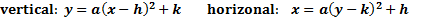Examples:

1. Find the equation of this parabola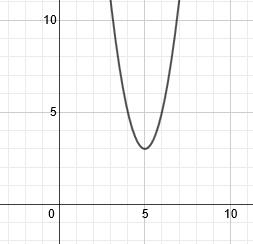This is a vertical parabola, so we are using the pattern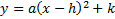Our vertex is (5, 3), so we will substitute those numbers in for h and k: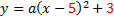Now we must choose a point to substitute in. You can choose any point on the parabola except the vertex. Let's use (4, 5). We'll substitute 4 in for x and 5 for y.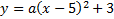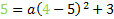Now we'll solve for a: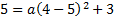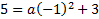5 = a(1) + 3

2 = a

To finish, we rewrite the pattern with h, k, and a:2. Find the equation of the parabola: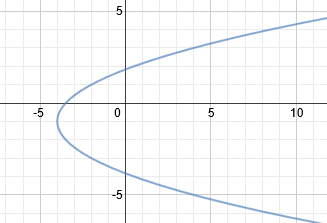This is a vertical parabola, so we are using the pattern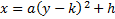Our vertex is (-4, -1), so we will substitute those numbers in for h and k: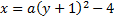Now we must choose a point to substitute in. You can choose any point on the parabola except the vertex. Let's use (4, 3). We'll substitute 4 in for x and 3 for y.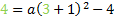Now we'll solve for a: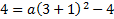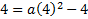4 = a(16) - 4

8 = 16a

1/2 = a

To finish, we rewrite the pattern with h, k, and a: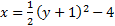Practice: Find the equation of these parabolas.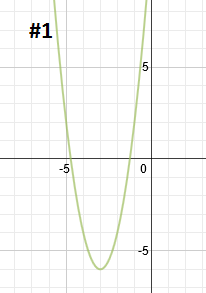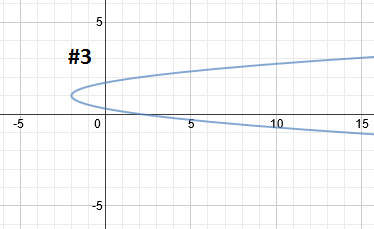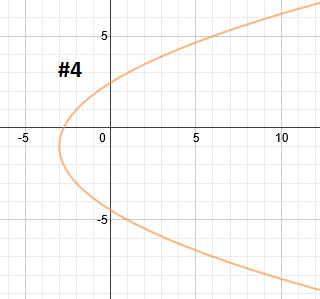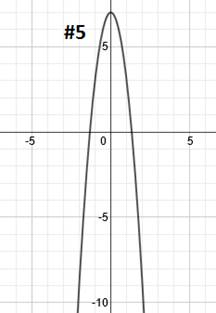Answers: 1)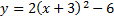2)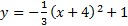3)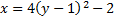4)5)Related Links: Math Fractions Factors